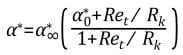## Fluids

•trippleD
Subscriber

Hello,

i would like to understand how the different turbulence models are working.Right now, i"m dealing with the Standard k-omega model. There is an option to do a low Reynolds-correction by using the named model. So the first question is the following:

What is a low Reynolds-number? Where is the limit of the definition of low Reynolds-numbers ? Is it the typical value of 2300?

The manual of fluent tells that the correction will be done by a damping coefficient. You can see the definiton below:I would like to understand these formulation as well. Where is it from and what does it do exactly?

I have another question. In the equations for k-omega or k-epsilon, you have expressions like G_k, G_b etc.. Are these expressions always used or does it depend on the application case ?

And there are also expression for S, called user-defind source terms. Does anyone have an example? i couldn't imagine anything.

trippled

•Rob
Ansys Employee

Please review the relevant sections of the manual, this may lead to the Theory Guide and most models will then have references for you to follow up on.

A User Defined Source is when we add something extra into a model using a source term, a typical example may be when we add energy (heat) to mimic a flame but don't want to model the combustion.

•trippleD
Subscriber

You can find it here:

https://www.sharcnet.ca/Software/Ansys/16.2.3/en-us/help/flu_th/flu_th_sec_turb_kw_std.html at 4.4.1.3.1. Low-Reynolds Number Correction.

How can I enable the user-defined source terms?

•Rob
Ansys Employee

I can find the up to date version through the Help function in Workbench & Fluent too: the Sharcnet links are about 5 years out of date.

Source terms can be found in the Cell zone conditions.

•trippleD
Subscriber

I use the Sharcnet links because i don"t know how to link the help function of Workbench. But the definition of alpha doesn"t change. Do you have an answer for my other questions?

I would like to add another thing; In the k-omega model, you have one quation for k and one for omega. In each equation, there are the coefficient i and j which go from 1 to 3. Just have a look at the equation for k. The value for i is 1 and j is running from 1 to 3. So all in all, i have 9 equation for k? Is it right?

•Rob
Ansys Employee

Read the documentation, and then review the references. Then let us know your findings.

•trippleD
Subscriber

I have read the documentation. If it had been clearly explained there, I wouldn't have asked the questions.

•Rob
Ansys Employee

And the references?

•trippleD
Subscriber

How do i review the reference? It is in theory guide-turbulence-standard, bsl and SST k-omega models-standard k-omega model-low reynolds number correction.

•Rob
Ansys Employee

The Low Re correction is in 4.4.1.3.1 of the 2019R2 Theory Guide. And this covers what it does, and states the alpha value for high Re flow. If we don't explain further it may be covered in the references to the k-w model: it's not something I have any additional information on, so your next step is the k-w reference(s) that will be in the Theory Guide.

•TateCollins
Subscriber
Hi Rob, I am having a similar issue trying to understand the meaning of the Low-Re corrections theoretically. I've tried to find the section you referenced above as 4.4.1.3.1 in the Theory Guide, however after the 3rd number I'm at the ?4.4.1 Standard k- epsilon Model? page but I am not sure where to go for the ?3.1 bit.nPlease could you provide a URL for the part of the Theory Guide you are referring to please.nCheers.n
•DrAmine
Ansys Employee
Hi All,nnLow-RE correction have been added to match DNS . data. They are of cosmetic nature: it is not required to enable them. They have been proposed by Wilcox.n
•Rob
Ansys Employee
https://ansyshelp.ansys.com/account/Secured?returnurl=/Views/Secured/corp/v194/flu_th/flu_th_sec_turb_kw_std.html#x1-1310005.6 n